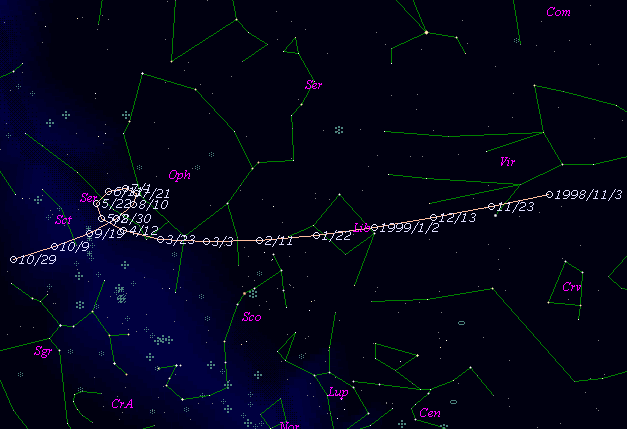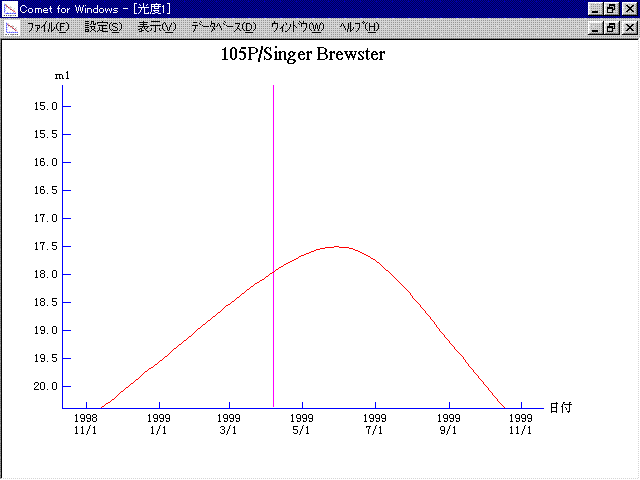# \$B%7%s%,!

105P/Singer Brewster (1999)###\$B50F;MWAG(B

```   The following improved orbital elements by Kenji Muraoka, are
from 57 observations 1986 to 1999, perturbations by 9 Plantes,
Moon and 5 minor planets were taken into account.
The mean residual is +/- 0.87 arc seconds.

Epoch  =  1999 Apr. 12.0  TT       JDT = 2451280.5
T  =  1999 Apr.  6.44977       +/- 0.00024 (m.e.) TT
Peri. =   46.80301                +/- 0.00020
Node  =  192.54586                +/- 0.00018   (2000.0)
Incl. =    9.18784                +/- 0.00004
q  =    2.0323716              +/- 0.0000010 AU
e  =    0.4127571              +/- 0.0000003
a  =    3.4608704              +/- 0.0000007 AU
n  =    0.15308269             +/- 0.00000005
P  =    6.438                  +/- 0.0000020  years
```

###\$B@1?^(B###\$B8wEYJQ2=(B

```        m1 = 12.5 + 5 log\$B&\$(B + 15.0 log r
```##### \$B50F;MWAG\$OB<2,7r<#;a\$N7W;;\$K\$h\$k\$b\$N\$G\$9!#(B \$B@1?^\$O(B StellaNavigator Ver.2.0 for Windows (\$B%"%9%H%m%"!<%D(B \$BJTCx(B / \$B%"%9%-!<=PHG6I4)(B) \$B\$G:n@.\$7\$?\$b\$N\$G\$9!#(B \$B8wEY%0%i%U\$O(BComet for Windows\$B\$G:n@.\$7\$?\$b\$N\$G\$9!#(B